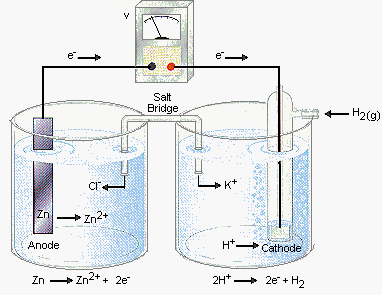Practice Problem 7

Write the line notation for the cell shown in the figure below.Answer

The cell is based on the following half-reactions.

 Oxidation: ZnZn2+ + 2 e- Reduction: 2 H+ + 2 e-H2

Since we list the reactants before the products in a half-cell, we write the following line notation for the anode.

 anode: Zn | Zn2+(1.0 M)

The line notation for the cathode has to indicate that H+ ions are reduced to H2 gas on a platinum metal surface. This can be done as follows.

 cathode: H+(1.0 M) | H2(1 atm) | Pt

Since line notation is read in the direction in which electrons flow, we place the notation for the anode before the cathode, as follows.

 Zn | Zn2+(1.0 M) || H+(1.0 M) | H2(1 atm) | Pt anode cathode